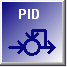PID Proportional-Integral-Derivative Control

Proportional-Integral-Derivative Control (PID) function blocks implement a standard PID control algorithm.

PID definition parameters

 Parameter Description Name Function block name, see common parameters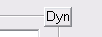DYN dynamic option, see common parameters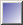/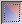Mixed inputs option, see common parameters Input The measured value or (process value) that is to be controlled. Set Point The target value (set point) that the control block is striving to attain. Control parameters Proportional Control's Proportional setting. Integral Control's Integral setting. Derivative Control's Derivative setting. Deadband Increasing/Decreasing If the error value is less than this value the control will not change its output. This can help prevent unnecessary cycling. There are two separate parameters which are applied depending on whether the output would be increasing or decreasing. Ramp Rate Limits the rate at which the output is allowed to change, the value is expressed as % per second. For example, if set at 20 a step PID output change from 0 to 100% would require 5 seconds to ramp up to 100%. Enable & Ramp Time If defined allows a digital input block to temporarily enable or disable the PID algorithm. When disabled the output goes to zero also it becomes possible to set the output value directly, via MBus_ioFlash or Modbus. When enabled the output will ramp to its required value over the entered "Ramp Time" interval.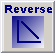/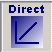Toggles between Reverse and Direct acting control mode (push to switch between modes): - In Reverse mode, the PID response is in inversely proportional with the weighted sum of the control parameters, heating application for example. - In Direct mode, the PID response is in direct proportion with the weighted sum of the control parameters, cooling application for example. This parameter can also be dynamically controlled by another block in the MBus_ioFlash program.

PID Control Algorithm Primer

A proportional–integral–derivative controller (PID controller) attempts to correct the error between a measured process variable and a desired set point by calculating and then outputting a corrective action that can adjust the process accordingly.

The PID controller algorithm involves three separate parameters; the Proportional, the Integral and Derivative values. The Proportional value determines the reaction to the current error, the Integral determines the reaction based on the sum of recent errors and the Derivative determines the reaction to the rate at which the error has been changing. The weighted sum of these three actions is used to adjust the process via a control element such as the position of a control valve or the power supply of a heating element.

By "tuning" the three constants in the PID controller algorithm the PID can provide control action designed for specific process requirements. The response of the controller can be described in terms of the responsiveness of the controller to an error, the degree to which the controller overshoots the setpoint and the degree of system oscillation. Note that the use of the PID algorithm for control does not guarantee optimal control of the system.

Some applications may require using only one or two modes to provide the appropriate system control. This is achieved by setting the gain of undesired control outputs to zero. A PID controller will be called a PI, PD, P or I controller in the absence of the respective control actions. PI controllers are particularly common, since derivative action is very sensitive to measurement noise, and the absence of an integral value prevents the system from reaching its target value due to the control action.